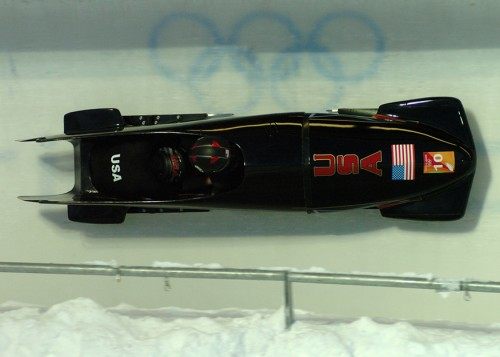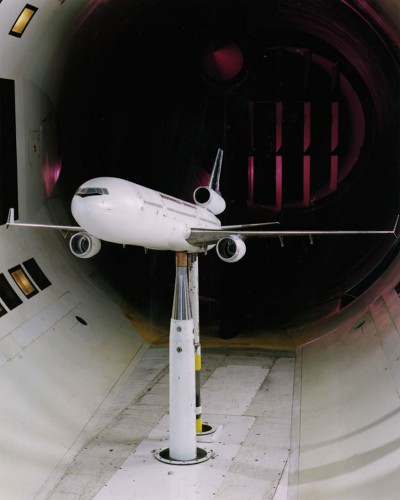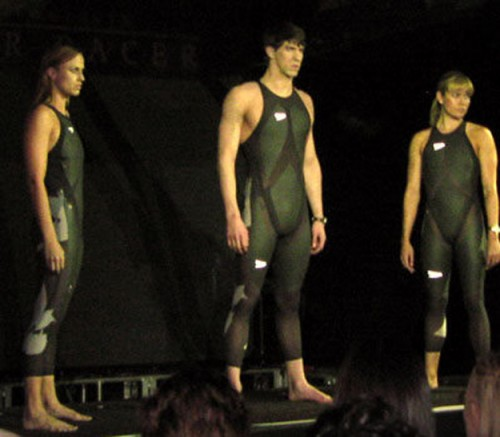## Drag Forces

### Learning Objectives

By the end of this section, you will be able to:

• Express mathematically the drag force.
• Discuss the applications of drag force.
• Define terminal velocity.
• Determine the terminal velocity given mass.

Another interesting force in everyday life is the force of drag on an object when it is moving in a fluid (either a gas or a liquid). You feel the drag force when you move your hand through water. You might also feel it if you move your hand during a strong wind. The faster you move your hand, the harder it is to move. You feel a smaller drag force when you tilt your hand so only the side goes through the air—you have decreased the area of your hand that faces the direction of motion. Like friction, the drag force always opposes the motion of an object. Unlike simple friction, the drag force is proportional to some function of the velocity of the object in that fluid. This functionality is complicated and depends upon the shape of the object, its size, its velocity, and the fluid it is in. For most large objects such as bicyclists, cars, and baseballs not moving too slowly, the magnitude of the drag force FD is found to be proportional to the square of the speed of the object. We can write this relationship mathematically as $F_{\text{D}}\propto{v}^2\\$. When taking into account other factors, this relationship becomes $F_{\text{D}}=\frac{1}{2}\text{C}\rho{A}v^2\\$, where C is the drag coefficient, A is the area of the object facing the fluid, and ρ is the density of the fluid. (Recall that density is mass per unit volume.) This equation can also be written in a more generalized fashion as FDbv2, where b is a constant equivalent to 0.5CρA. We have set the exponent n for these equations as 2 because, when an object is moving at high velocity through air, the magnitude of the drag force is proportional to the square of the speed. As we shall see in a few pages on fluid dynamics, for small particles moving at low speeds in a fluid, the exponent n is equal to 1.

### Drag Force

Drag force FD is found to be proportional to the square of the speed of the object. Mathematically

$F_{\text{D}}\propto{v}^2\\$

$F_{\text{D}}=\frac{1}{2}\text{C}\rho{A}v^2\\$,

where C is the drag coefficient, A is the area of the object facing the fluid, and ρ is the density of the fluid.

Athletes as well as car designers seek to reduce the drag force to lower their race times. (See Figure 1). “Aerodynamic” shaping of an automobile can reduce the drag force and so increase a car’s gas mileage.Figure 1. From racing cars to bobsled racers, aerodynamic shaping is crucial to achieving top speeds. Bobsleds are designed for speed. They are shaped like a bullet with tapered fins. (credit: U.S. Army, via Wikimedia Commons)

The value of the drag coefficient, C , is determined empirically, usually with the use of a wind tunnel. (See Figure 2).Figure 2. NASA researchers test a model plane in a wind tunnel. (credit: NASA/Ames)

The drag coefficient can depend upon velocity, but we will assume that it is a constant here. Table 1 lists some typical drag coefficients for a variety of objects. Notice that the drag coefficient is a dimensionless quantity. At highway speeds, over 50% of the power of a car is used to overcome air drag. The most fuel-efficient cruising speed is about 70–80 km/h (about 45–50 mi/h). For this reason, during the 1970s oil crisis in the United States, maximum speeds on highways were set at about 90 km/h (55 mi/h).

Table 1. Drag Coefficient Values Typical values of drag coefficient C.
OBJECT C
Airfoil 0.05
Toyota Camry 0.28
Ford Focus 0.32
Honda Civic 0.36
Ferrari Testarossa 0.37
Dodge Ram pickup 0.43
Sphere 0.45
Hummer H2 SUV 0.64
Skydiver (feet first) 0.70
Bicycle 0.90
Skydiver (horizontal) 1.0
Circular flat plate 1.12Figure 3. Body suits, such as this LZR Racer Suit, have been credited with many world records after their release in 2008. Smoother “skin” and more compression forces on a swimmer’s body provide at least 10% less drag. (credit: NASA/Kathy Barnstorff)

Substantial research is under way in the sporting world to minimize drag. The dimples on golf balls are being redesigned as are the clothes that athletes wear. Bicycle racers and some swimmers and runners wear full bodysuits. Australian Cathy Freeman wore a full body suit in the 2000 Sydney Olympics, and won the gold medal for the 400 m race. Many swimmers in the 2008 Beijing Olympics wore (Speedo) body suits; it might have made a difference in breaking many world records (See Figure 3). Most elite swimmers (and cyclists) shave their body hair. Such innovations can have the effect of slicing away milliseconds in a race, sometimes making the difference between a gold and a silver medal. One consequence is that careful and precise guidelines must be continuously developed to maintain the integrity of the sport.

Some interesting situations connected to Newton’s second law occur when considering the effects of drag forces upon a moving object. For instance, consider a skydiver falling through air under the influence of gravity. The two forces acting on him are the force of gravity and the drag force (ignoring the buoyant force). The downward force of gravity remains constant regardless of the velocity at which the person is moving. However, as the person’s velocity increases, the magnitude of the drag force increases until the magnitude of the drag force is equal to the gravitational force, thus producing a net force of zero. A zero net force means that there is no acceleration, as given by Newton’s second law. At this point, the person’s velocity remains constant and we say that the person has reached his terminal velocity (vt). Since FD is proportional to the speed, a heavier skydiver must go faster for FD to equal his weight. Let’s see how this works out more quantitatively.

At the terminal velocity, $F_{\text{net}}=mg-F_{\text{D}}=ma=0\\$. Thus, $mg=F_{\text{D}}\\$. Using the equation for drag force, we have $mg=\frac{1}{2}\rho{CAv}^2\\$. Solving for the velocity, we obtain $v=\sqrt{\frac{2mg}{\rho{CA}}}\\$.

Assume the density of air is ρ = 1.21 kg/m3. A 75-kg skydiver descending head first will have an area approximately A = 0.18 m2 and a drag coefficient of approximately C=0.70. We find that

$\begin{array}{lll}v& =& \sqrt{\frac{2\left(\text{75 kg}\right)\left(9\text{.80 m}{\text{/s}}^{2}\right)}{\left(1\text{.}\text{21 kg}{\text{/m}}^{3}\right)\left(0\text{.}\text{70}\right)\left(\text{0.18}{\text{m}}^{2}\right)}}\\ & =& \text{98 m/s}\\ & =& \text{350 km/h}\text{.}\end{array}\\$

This means a skydiver with a mass of 75 kg achieves a maximum terminal velocity of about 350 km/h while traveling in a pike (head first) position, minimizing the area and his drag. In a spread-eagle position, that terminal velocity may decrease to about 200 km/h as the area increases. This terminal velocity becomes much smaller after the parachute opens.

### Take-Home Experiment

This interesting activity examines the effect of weight upon terminal velocity. Gather together some nested coffee filters. Leaving them in their original shape, measure the time it takes for one, two, three, four, and five nested filters to fall to the floor from the same height (roughly 2 m). (Note that, due to the way the filters are nested, drag is constant and only mass varies.) They obtain terminal velocity quite quickly, so find this velocity as a function of mass. Plot the terminal velocity v versus mass. Also plot v2 versus mass. Which of these relationships is more linear? What can you conclude from these graphs?

### Example 1. A Terminal Velocity

Find the terminal velocity of an 85-kg skydiver falling in a spread-eagle position.

#### Strategy

At terminal velocity, Fnet = 0. Thus the drag force on the skydiver must equal the force of gravity (the person’s weight). Using the equation of drag force, we find $mg=\frac{1}{2}\rho{CAv}^2\\$.

Thus the terminal velocity vt can be written as $v_{\text{t}}\sqrt{\frac{2mg}{\rho{CA}}}\\$.

#### Solution

All quantities are known except the person’s projected area. This is an adult (82 kg) falling spread eagle. We can estimate the frontal area as A = (2 m)(0.35 m) = 0.70 m2.

Using our equation for v, we find that

$\begin{array}{lll}{v}_{\text{t}}& =& \sqrt{\frac{2\left(\text{85}\text{kg}\right)\left(9.80{\text{m/s}}^{2}\right)}{\left(1.21{\text{kg/m}}^{3}\right)\left(1.0\right)\left(0.70{\text{m}}^{2}\right)}}\\ & =& \text{44 m/s.}\end{array}\\$

#### Discussion

This result is consistent with the value for vt mentioned earlier. The 75-kg skydiver going feet first had a v = 98 m/s. He weighed less but had a smaller frontal area and so a smaller drag due to the air.

The size of the object that is falling through air presents another interesting application of air drag. If you fall from a 5-m high branch of a tree, you will likely get hurt—possibly fracturing a bone. However, a small squirrel does this all the time, without getting hurt. You don’t reach a terminal velocity in such a short distance, but the squirrel does.

The following interesting quote on animal size and terminal velocity is from a 1928 essay by a British biologist, J.B.S. Haldane, titled “On Being the Right Size.”

To the mouse and any smaller animal, [gravity] presents practically no dangers. You can drop a mouse down a thousand-yard mine shaft; and, on arriving at the bottom, it gets a slight shock and walks away, provided that the ground is fairly soft. A rat is killed, a man is broken, and a horse splashes. For the resistance presented to movement by the air is proportional to the surface of the moving object. Divide an animal’s length, breadth, and height each by ten; its weight is reduced to a thousandth, but its surface only to a hundredth. So the resistance to falling in the case of the small animal is relatively ten times greater than the driving force.

The above quadratic dependence of air drag upon velocity does not hold if the object is very small, is going very slow, or is in a denser medium than air. Then we find that the drag force is proportional just to the velocity. This relationship is given by Stokes’ law, which states that Fs = 6πrηv, where r is the radius of the object, η is the viscosity of the fluid, and v is the object’s velocity.

### Stokes’ Law

Fs = 6πrηv, where r is the radius of the object, η is the viscosity of the fluid, and v is the object’s velocity.Figure 4. Geese fly in a V formation during their long migratory travels. This shape reduces drag and energy consumption for individual birds, and also allows them a better way to communicate. (credit: Julo, Wikimedia Commons)

Good examples of this law are provided by microorganisms, pollen, and dust particles. Because each of these objects is so small, we find that many of these objects travel unaided only at a constant (terminal) velocity. Terminal velocities for bacteria (size about 1 μm) can be about 2 μm/s. To move at a greater speed, many bacteria swim using flagella (organelles shaped like little tails) that are powered by little motors embedded in the cell. Sediment in a lake can move at a greater terminal velocity (about 5μ m/s), so it can take days to reach the bottom of the lake after being deposited on the surface.

If we compare animals living on land with those in water, you can see how drag has influenced evolution. Fishes, dolphins, and even massive whales are streamlined in shape to reduce drag forces. Birds are streamlined and migratory species that fly large distances often have particular features such as long necks. Flocks of birds fly in the shape of a spear head as the flock forms a streamlined pattern (see Figure 4). In humans, one important example of streamlining is the shape of sperm, which need to be efficient in their use of energy.

### Galileo’s Experiment

Galileo is said to have dropped two objects of different masses from the Tower of Pisa. He measured how long it took each to reach the ground. Since stopwatches weren’t readily available, how do you think he measured their fall time? If the objects were the same size, but with different masses, what do you think he should have observed? Would this result be different if done on the Moon?

## PhET Explorations: Masses & Springs

A realistic mass and spring laboratory. Hang masses from springs and adjust the spring stiffness and damping. You can even slow time. Transport the lab to different planets. A chart shows the kinetic, potential, and thermal energy for each spring.

## Section Summary

• Drag forces acting on an object moving in a fluid oppose the motion. For larger objects (such as a baseball) moving at a velocity v in air, the drag force is given by ${F}_{\text{D}}=\frac{1}{2}\text{C}\rho{Av}^{2}\\$, where C is the drag coefficient (typical values are given in Table 1), A is the area of the object facing the fluid, and $\rho\\$ is the fluid density.
• For small objects (such as a bacterium) moving in a denser medium (such as water), the drag force is given by Stokes’ law, ${F}_{\text{s}}=6\pi\eta{rv}\\$, where r is the radius of the object, η is the fluid viscosity, and v is the object’s velocity.

### Conceptual Questions

1. Athletes such as swimmers and bicyclists wear body suits in competition. Formulate a list of pros and cons of such suits.
2. Two expressions were used for the drag force experienced by a moving object in a liquid. One depended upon the speed, while the other was proportional to the square of the speed. In which types of motion would each of these expressions be more applicable than the other one?
3. As cars travel, oil and gasoline leaks onto the road surface. If a light rain falls, what does this do to the control of the car? Does a heavy rain make any difference?
4. Why can a squirrel jump from a tree branch to the ground and run away undamaged, while a human could break a bone in such a fall?

### Problems & Exercises

1. The terminal velocity of a person falling in air depends upon the weight and the area of the person facing the fluid. Find the terminal velocity (in meters per second and kilometers per hour) of an 80.0-kg skydiver falling in a pike (headfirst) position with a surface area of 0.140 m2.
2. A 60-kg and a 90-kg skydiver jump from an airplane at an altitude of 6000 m, both falling in the pike position. Make some assumption on their frontal areas and calculate their terminal velocities. How long will it take for each skydiver to reach the ground (assuming the time to reach terminal velocity is small)? Assume all values are accurate to three significant digits.
3. A 560-g squirrel with a surface area of 930 cm2 falls from a 5.0-m tree to the ground. Estimate its terminal velocity. (Use a drag coefficient for a horizontal skydiver.) What will be the velocity of a 56-kg person hitting the ground, assuming no drag contribution in such a short distance?
4. To maintain a constant speed, the force provided by a car’s engine must equal the drag force plus the force of friction of the road (the rolling resistance). (a) What are the magnitudes of drag forces at 70 km/h and 100 km/h for a Toyota Camry? (Drag area is 0.70 m2) (b)What is the magnitude of drag force at 70 km/h and 100 km/h for a Hummer H2? (Drag area is 2.44 m2) Assume all values are accurate to three significant digits.
5. By what factor does the drag force on a car increase as it goes from 65 to 110 km/h?
6. Calculate the speed a spherical rain drop would achieve falling from 5.00 km (a) in the absence of air drag (b) with air drag. Take the size across of the drop to be 4 mm, the density to be 1.00 × 103 kg/m3, and the surface area to be πr2.
7. Using Stokes’ law, verify that the units for viscosity are kilograms per meter per second.
8. Find the terminal velocity of a spherical bacterium (diameter 2.00 μ m) falling in water. You will first need to note that the drag force is equal to the weight at terminal velocity. Take the density of the bacterium to be 1.10 × 103 kg/m3.
9. Stokes’ law describes sedimentation of particles in liquids and can be used to measure viscosity. Particles in liquids achieve terminal velocity quickly. One can measure the time it takes for a particle to fall a certain distance and then use Stokes’ law to calculate the viscosity of the liquid. Suppose a steel ball bearing (density 7.8 × 103 kg/m3, diameter 3.0 mm) is dropped in a container of motor oil. It takes 12 s to fall a distance of 0.60 m. Calculate the viscosity of the oil.

## Glossary

drag force: FD, found to be proportional to the square of the speed of the object; mathematically ${F}_{\text{D}}\propto {v}^{\text{2}}\\$, ${F}_{\text{D}}=\frac{1}{2}C\rho{Av}^{2}\\$, where C is the drag coefficient, A is the area of the object facing the fluid, and $\rho$ is the density of the fluid

Stokes’ law: ${F}_{s}=6\pi{r}\eta{v}\\$ , where is the radius of the object, η is the viscosity of the fluid, and v is the object’s velocity

### Selected Solutions to Problems & Exercises

1. 115 m/s; 414 km/hr

3. 25 m/s; 9.9 m/s

5. 2.9

7. $\left[\eta \right]=\frac{\left[{F}_{\text{s}}\right]}{\left[r\right]\left[v\right]}=\frac{\text{kg}\cdot {\text{m/s}}^{2}}{\text{m}\cdot \text{m/s}}=\frac{\text{kg}}{\text{m}\cdot \text{s}}\\$

9. 0.76 kg/m·s